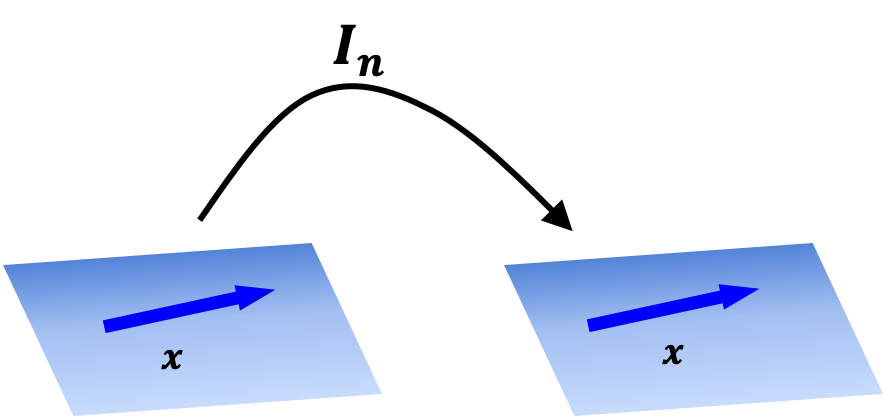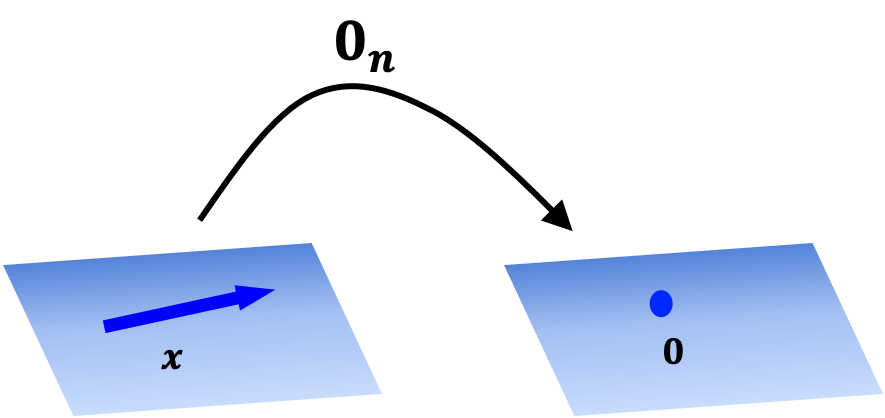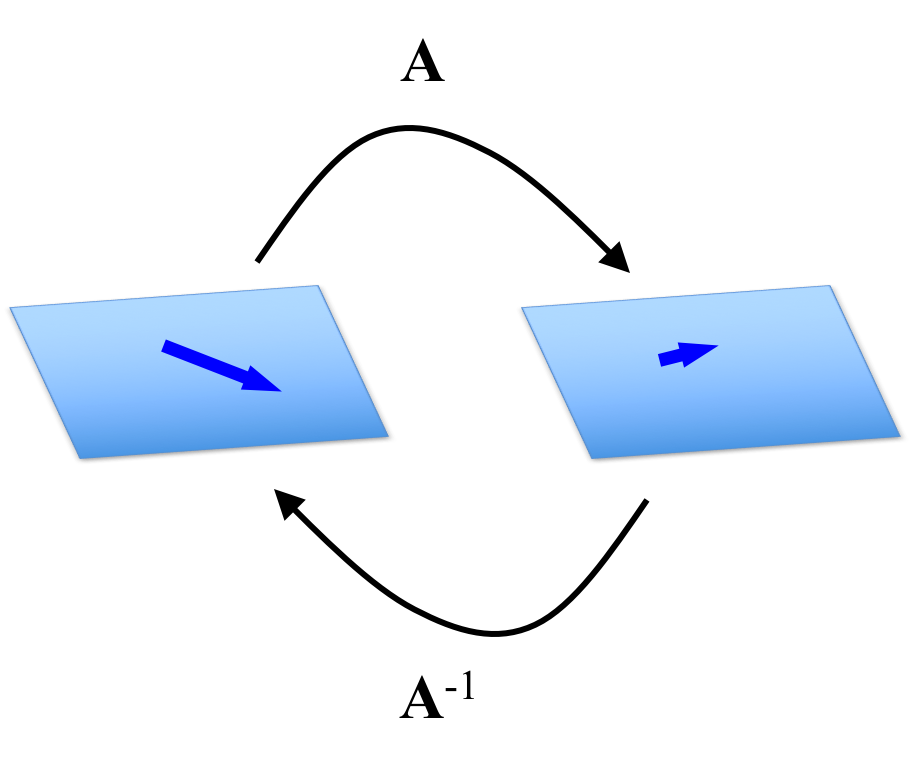# Matrices as functions

Published:

At the core of linear algebra is the idea that matrices represent functions. In this post, we’ll look at a few common, elementary functions and discuss their corresponding matrices.

## Introduction

Recall that the definition of matrix-vector multiplication enables us treat matrices as functions in the following sense: if we hold a matrix $\boldsymbol{A} \in \mathbb{R}^{m \times n}$ as fixed, this matrix maps vectors in $\mathbb{R}^n$ to vectors in $\mathbb{R}^m$. That is, we can define a function $T : \mathbb{R}^n \rightarrow \mathbb{R}^m$ as:

$T(\boldsymbol{x}) := \boldsymbol{A}\boldsymbol{x}$

In this post, we’ll look at a few common, elementary functions and discuss their corresponding matrices.

## The identity matrix defines the identity function

Recall an identity function $f$ for a set $S$ is the function $f(x) := x$ for all $x \in S$. In the context of a function $T$ over a vector space $\mathbb{R}^n$, the identity function $T(\boldsymbol{x}) := \boldsymbol{x}$ for all $\boldsymbol{x} \in \mathbb{R}^n$ is defined using the identity matrix for $\mathbb{R}^n$. The identity matrix for $\mathbb{R}^n$, denoted $\boldsymbol{I}_n$ (or simply $\boldsymbol{I}$ if the dimensionality is implied by the context), is a square matrix of all zeros except for ones along the diagonal:

Definition 1 (Identity matrix): Each real-valued, Euclidean vector space $\mathbb{R}^n$ is associated with an identity matrix, denoted $\boldsymbol{I}_{n \times n}$ (or simply $\boldsymbol{I}$ if the dimensionality is implied by the context), which is a square matrix consisting of zeros in the off-diagonal entries and ones along the diagonal.

For example, the identity matrix for $\mathbb{R}^3$ is defined as

$\boldsymbol{I}_3 := \begin{bmatrix} 1 & 0 & 0 \\ 0 & 1 & 0 \\ 0 & 0 & 1 \end{bmatrix}$

It can easily be shown that applying matrix-vector multiplication using an identity matrix $\boldsymbol{I}_n$ with any vector $\boldsymbol{x} \in \mathbb{R}^n$ will result in the same vector $\boldsymbol{x}$. Thus, a function $T(\boldsymbol{x}) := \boldsymbol{I}\boldsymbol{x}$ is the identity function with domain $\mathbb{R}^n$. This is depicted schematically below:## The zero matrix defines the zero function

Recall a zero-function $f$ for a set $S$ is the function $f(x) := 0$ for all $x \in S$. In the context of a function $T$ over a vector space $\mathbb{R}^n$, the zero function $T(\boldsymbol{x}) := \boldsymbol{0}$ for all $\boldsymbol{x} \in \mathbb{R}^n$ is defined using the zero matrix for $\mathbb{R}^n$. The zero matrix for $\mathbb{R}^n$, denoted $\boldsymbol{0}_n$ is a square matrix of all zeros:

Definition 2 (Zero matrix): Each real-valued, Euclidean vector space $\mathbb{R}^n$ is associated with a zero matrix, denoted $\boldsymbol{0}_{n \times n}$, which is a square matrix consisting of all zeros.

For example, the zero matrix for $\mathbb{R}^3$ is defined as

$\boldsymbol{0}_3 := \begin{bmatrix} 0 & 0 & 0\\ 0 & 0 & 0 \\ 0 & 0 & 0 \end{bmatrix}$

It can easily be shown that applying matrix-vector multiplication using an identity matrix $\boldsymbol{0}_n$ with any vector $\boldsymbol{x} \in \mathbb{R}^n$ will result in the zero vector $\boldsymbol{0}$. Thus, a function $T(\boldsymbol{x}) := \boldsymbol{0}_n\boldsymbol{x}$ is the zero function for domain $\mathbb{R}^n$. This is depicted schematically below:## An inverse matrix defines an inverse function

Let’s say we have some function $T(\boldsymbol{x}) := \boldsymbol{Ax}$. Let’s say there exists a matrix $\boldsymbol{C}$ such that the function $F(\boldsymbol{x}) := \boldsymbol{Cx}$ is the inverse function of $T$. That is, $F = T^{-1}$ where

$T^{-1}(T(\boldsymbol{x})) = \boldsymbol{x}$

If this is the case, then we call $\boldsymbol{C}$ the inverse matrix of $\boldsymbol{A}$. Usually, we will denote this inverse matrix $\boldsymbol{C}$ as $\boldsymbol{A}^{-1}$. This is depicted in the figure below:Not every matrix has an inverse. Matrices that have inverses are a special category of matrix called invertible matrices and have special properties, which we will discuss in a future post.

Tags: